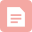# 2022-01-13每日刷题打卡

## 飞书——每日一题

#### 678. 有效的括号字符串

• 可以被视为单个右括号 ) ，或单个左括号 ( ，或一个空字符串。
一个空字符串也被视为有效字符串。
示例 1:

class Solution {
public:
bool checkValidString(string s) {
stack<int>sta;
stack<int>star;
int n=s.size();
for(int i=0;i<n;i++)
{
char c=s[i];
if(c=='(')sta.push(i);
else if(c==')')
{
if(sta.size())sta.pop();
else if(star.size())star.pop();
else return false;
}
else star.push(i);
}
while(sta.size())
{
if(star.size()&&sta.top()<star.top())
{
sta.pop();
star.pop();
}
else return false;
}
return true;
}
};


## AcWing——y总算法课

1≤n≤500
1≤m≤10^5

#### 输入样例：

3 3
1 2 2
2 3 1
1 3 4


#### 输出样例：

3

#include<iostream>
using namespace std;
#include<string.h>

const int N=510;
int g[N][N],dist[N],n,m;
bool flag[N];

int dijkstra()
{
memset(dist,0x3f,sizeof dist);
dist=0;
for(int i=0;i<n;i++)
{
int t=-1;
for(int j=1;j<=n;j++)
if(!flag[j]&&(t==-1||dist[t]>dist[j]))
t=j;
flag[t]=true;

for(int j=1;j<=n;j++)
{
dist[j]=min(dist[j],g[t][j]+dist[t]);
}
}
if(dist[n]==0x3f3f3f3f)return -1;
return dist[n];
}

int main()
{
cin>>n>>m;
memset(g,0x3f,sizeof g);
int a,b,c;
for(int i=1;i<=m;i++)
{
cin>>a>>b>>c;
g[a][b]=min(g[a][b],c);
}
cout<<dijkstra()<<endl;
return 0;
}


1≤n,m≤1.5×10^5

#### 输入样例：

3 3
1 2 2
2 3 1
1 3 4


#### 输出样例：

3

#include<iostream>
using namespace std;
#include<queue>
#include<string.h>

const int N=150010;
int idx,w[N],h[N],e[N],ne[N],n,m,dist[N];
bool flag[N];

typedef pair<int,int>PII;

{
e[idx]=b,w[idx]=c,ne[idx]=h[a],h[a]=idx++;
}

int dijkstra()
{
memset(dist,0x3f,sizeof dist);
dist=0;
priority_queue<PII,vector<PII>,greater<PII>>heap;
heap.push({0,1});
while(heap.size())
{
auto t=heap.top();
heap.pop();
int ver=t.second,distance=t.first;
if(flag[ver])continue;
flag[ver]=true;
for(int i=h[ver];i!=-1;i=ne[i])
{
int j=e[i];
if(dist[j]>distance+w[i])
{
dist[j]=distance+w[i];
heap.push({dist[j],j});
}
}
}
if(dist[n]==0x3f3f3f3f)return -1;
return dist[n];
}

int main()
{
int a,b,c;
memset(h,-1,sizeof h);
cin>>n>>m;
while(m--)
{
cin>>a>>b>>c;
}
cout<<dijkstra()<<endl;
return 0;
}


## 蓝桥杯——算法提高

#### 高精度加法

2 3
01
001

2

N,M<=10000

#include<iostream>
using namespace std;
#include<vector>

{
vector<int>C;
int t=0;
for(int i=0;i<A.size()||i<B.size();i++)
{
if(i<A.size())t+=A[i];
if(i<B.size())t+=B[i];
C.push_back(t%10);
t/=10;
}
if(t)C.push_back(1);
return C;
}

int main()
{
string a,b;
int n,m;
cin>>n>>m;
cin>>a>>b;
vector<int>A,B;
for(int i=a.size()-1;i>=0;i--)A.push_back(a[i]-'0');
for(int i=b.size()-1;i>=0;i--)B.push_back(b[i]-'0');

for(int i=C.size()-1;i>=0;i--)cout<<C[i];
return 0;
}博客
2022-01-03每日刷题打卡
01-0379博客
2022-04-01每日刷题打卡
04-014291博客
2022-01-02每日刷题打卡
01-02561博客
2022-01-01每日刷题打卡
01-01591博客
2022-01-26每日刷题打卡
01-265531博客
2022-01-19每日刷题打卡
01-19624博客
2022-01-16每日刷题打卡
01-16203博客
2022-01-28每日刷题打卡
01-281087博客
2022-01-30每日刷题打卡
01-30591博客
2022-01-12每日刷题打卡
01-1228博客
2022-01-06每日刷题打卡
01-0737博客
2022-01-20每日刷题打卡
01-20418博客
2022-01-29每日刷题打卡
01-2927博客
2022-01-27每日刷题打卡
01-27368博客
2022-01-09每日刷题打卡
01-09233博客

05-18160博客
ARC133 B - Dividing Subsequence
05-1834博客
GoLang之切片底层系列二(浅显学习)
05-1749博客

05-1785

### “相关推荐”对你有帮助么？

•非常没帮助
•没帮助
•一般
•有帮助
•非常有帮助¥2 ¥4 ¥6 ¥10 ¥20余额支付 (余额：-- )扫码支付获取中扫码支付点击重新获取扫码支付1.余额是钱包充值的虚拟货币，按照1:1的比例进行支付金额的抵扣。
2.余额无法直接购买下载，可以购买VIP、C币套餐、付费专栏及课程。余额充值